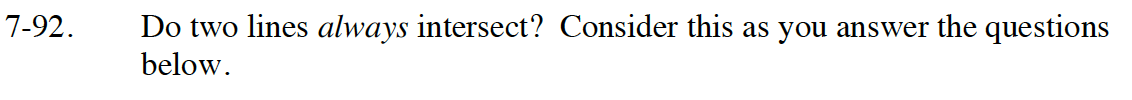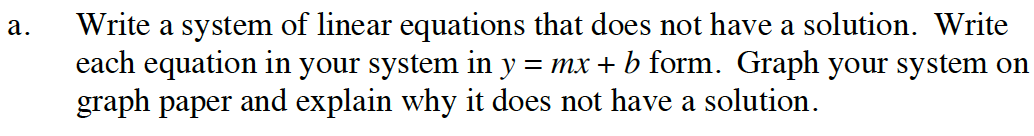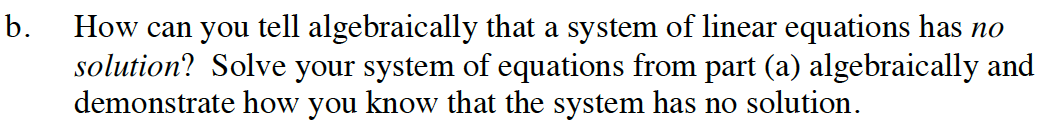### Home > CC4 > Chapter 7 > Lesson 7.2.1 > Problem7-92

7-92.
1. Do two lines always intersect? Consider this as you answer the questions below. Homework Help ✎

1. Write a system of linear equations that does not have a solution. Write each equation in your system in y = mx + b form. Graph your system on graph paper and explain why it does not have a solution..

2. How can you tell algebraically that a system of linear equations has no solution? Solve your system of equations from part (a) algebraically and demonstrate how you know that the system has no solution.Write a system of equations using two lines that have the same slope.

y = 2x + 1 and y = 2x − 3

Since the two lines have the same slope but different y-intercepts,
they will never intersect each other, hence there is no solution.Try solving 2x + 1 = 2x − 3 algebraically.

Since 1 ≠ −3, there is no solution to this system of equations.